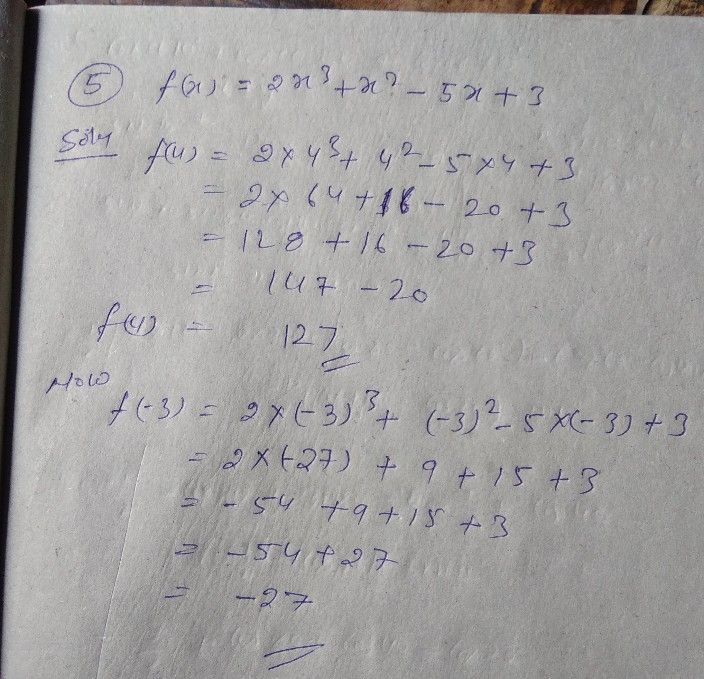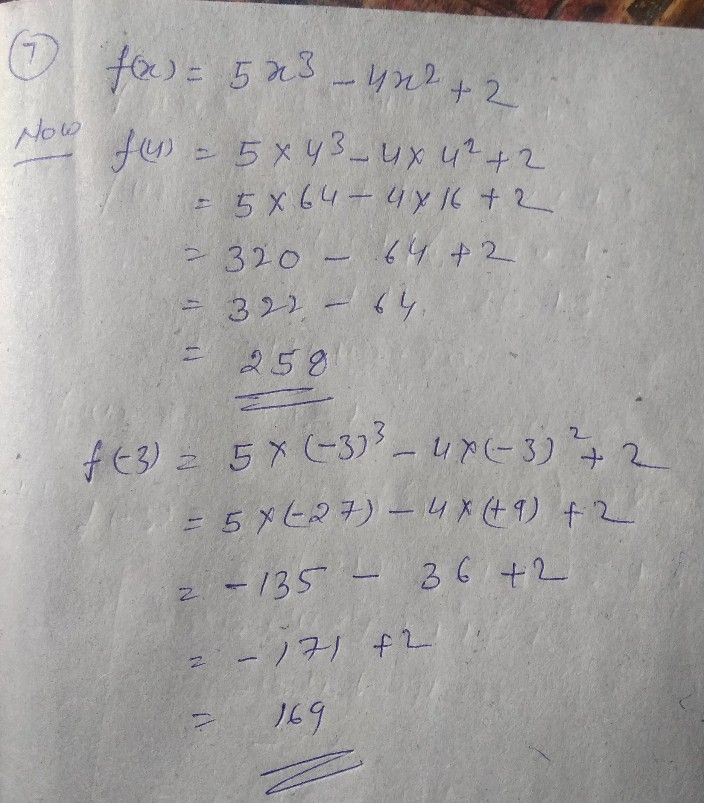Symbol
ProblemUse synthetic substitution to find $f\left(4\right)$ and $f\left(-3\right)$ for each function. $5.$ $f\left(x\right)=2x^{3}+x^{2}-5x+3$ $6.f\left(x\right)=3x^{3}-4x+2$ – $7.f\left(x\right)=5x^{3}-4x^{2}+2$ $8.f\left(x\right)=2x^{4}-4x^{3}+3x^{2}+x-6$ – $9.f\left(x\right)=5x^{4}+3x^{3}-4x^{2}-2x+4$ $10.f\left(x\right)=3x^{4}-2x^{3}-x^{2}+2x-5$ $11.f\left(x\right)=2x^{4}-4x^{3}-x^{2}-6x+3$ $12.f\left(x\right)=4x^{4}-4x^{3}+3x^{2}-2x-3$
10th-13th grade
Algebra
Search count: 133
SolutionQanda teacher - LaxmiStudent
what about the rest
or 7 atleastQanda teacher - Laxmi
okey I do it waitStudent
ok, thank you
please solve all parts of the questionQanda teacher - Laxmi
dear team Qanda dost not allow to solve more question at one time....they give me warining and say to banned me ....so you send it to my preffred questions id